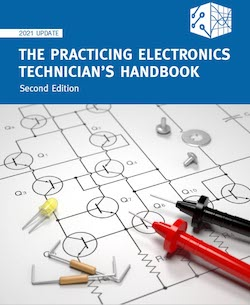### PRACTICING ELECTRONICS TECHNICIAN'S HANDBOOK Second Edition

Need to quickly find the formula for calculating resistance? Still need clarification about common characteristics of weighted number systems? Find these topics, and many more, in the Practicing Electronics Technician's Handbook Second Edition!

This handbook contains a wealth of useful day-to-day information and practical guidance for practicing electronics and electromechanical technicians and students. It is certain to become a valuable resource at home and at work.# Practicing Technician’s Handbook

### Key Features

• Over 60 pages of useful content, divided into three sections for easy reference: articles, key equation, and supplemental content
• Articles that clearly explain complex topics such as: math skills needed to solve simultaneous equations, common characteristics of weighted number systems, using the natural log function in circuit analysis, and converting electrical circuits to their series equivalent
• Over 80 commonly used formulas
• Supplemental content covers additional relevant topics, such as understanding electric charges, resistance, and RC circuits